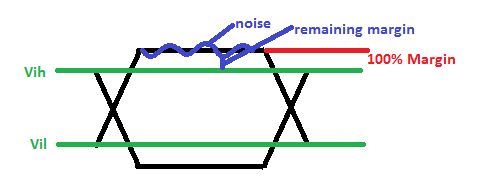# AR# 44394

## 描述

If I am targeting a 7 Series FPGA in the PlanAhead tool and performing SSN, how is the tool analyzing the amount of noise in the system compared to the ideal margin?

## 解决方案

VDH (voltage drive high) - Vih min (voltage input high) =  the logic 1 ideal margin.

Vil (voltage input low) - VDL (voltage drive low) = the logic 0 ideal margin.

Using a hammer 101010 pattern at the resonant frequency, the noise at the far end of the line is measured for logic 1 and logic 0.

The limit is the number of I/Os that can switch and still have remaining margin.

The remaining margin is the minimum of logic 1 ideal margin, logic1 noise, and logic 0 ideal margin, logic 0 noise.